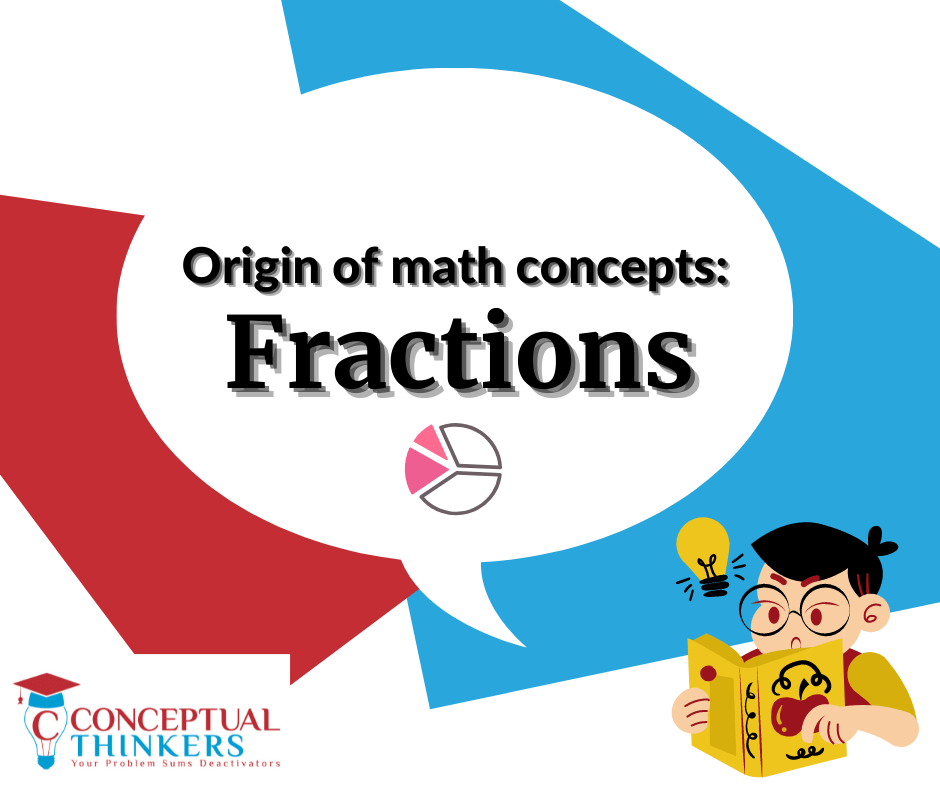# Origins of Math Concepts – FractionsDo you ever wonder how the mathematics concepts found in primary school textbooks came about? In the first topic of this series, we explore the origins of fractions and how the concept has evolved over the centuries.

## Egyptians – one of the early starters

One of the first uses of fractions was in 1800 BC by Egyptians, who expressed them as unit fractions based on the Egyptian numeration system, because they needed to find a way to divide food among the people. A unit fraction has a ‘1’ in the numerator and any positive number in the denominator (e.g. ½, ⅕).

For example, this is how they wrote 1/15:

Symbols were also used to show the different operations using fractions. For example, the addition of ⅕ and ⅓ could be shown like this:

If the man’s feet points to the right, it means addition and to the left would mean subtraction.

Some limitations of this system was that addition of repeated fractions weren’t allowed and it was also difficult to do more advanced calculations.

## Historical development by the Hindus

A closer representation of the modern day fraction could be traced back to the Hindus, who discovered it around A.D. 630. They used a writing system called brahmi, which consisted of nine symbols and a zero – similar to the number system used today. The fractions were written with numbers but without the bar, as shown below.

## Several other well-known contributions

The Arabs came up with the idea of putting a bar in between the top and the bottom number to separate them.

Later on, several Latin writers came up with the notion of numerator and denominator. In fact, the word fraction came from the Latin word ‘fractio’, which means to break.

For addition and subtraction of fractions, the common denominator was found using the product of both denominators until the sixteenth century. Later on, it was found using the lowest common multiple of both numbers.

The method of multiplication is similar to that of the present day. Interestingly for division in the past, the common multiple was also found. .Another way was through the use of cross-multiplication.

Today, fractions are mainly divide through the Keep-Change-Flip approach, where the division sign is changed to multiplication and the second fraction is flipped.

If your child finds fractions difficult or doesn’t want to do it, try showing them how fractions were used in the past and it may help to pique their interest in the topic. Here’s an example you could try with your child: